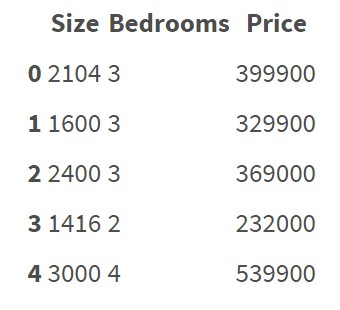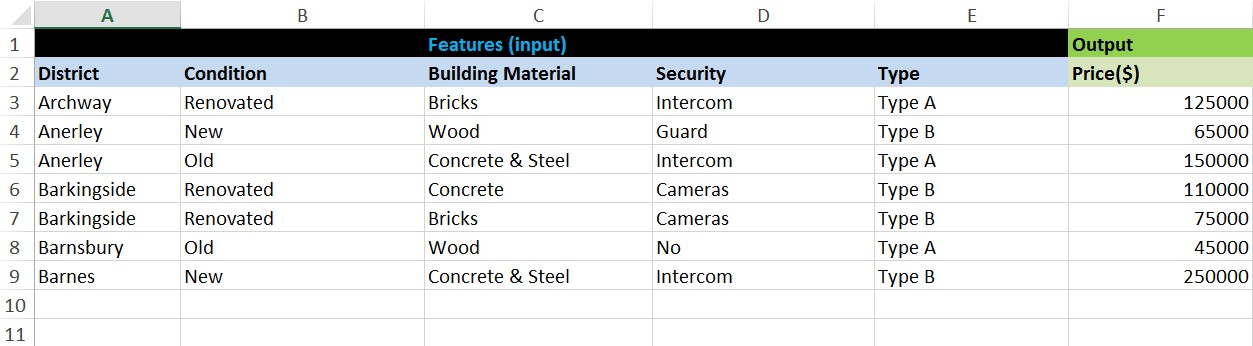2 views

# Regression algorithms seem to be working on features represented as numbers. For example:This dataset doesn't contain categorical features/variables. It's quite clear how to do regression on this data and predict the price.

But now I want to do regression analysis on data that contain categorical features:How can I do regression on this data? Do I have to transform all this string/categorical data to numbers manually? I mean if I have to create some encoding rules and according to that rules transform all data to numeric values. Is there any simple way to transform string data to numbers without having to create own encoding rules manually? Maybe there are some libraries in Python that can be used for that? Are there some risks that the regression model will be somehow incorrect due to "bad encoding"?

by (33.1k points)

In your problem, you have lots of categorical data. It is not suitable to train a precise machine learning model. To train a machine learning model efficiently, you need to convert categorical data to numbers.

To solve this problem, you can use One hot encoding to encode use words into numbers.

One hot encoding in scikit learn is an efficient way to implement this.

One Hot Encoder:

Encode categorical integer features as a one-hot numeric array. By default, the encoder derives the categories based on the unique values in each feature.

For example:

>>> from sklearn.preprocessing import OneHotEncoder

>>> enc = OneHotEncoder(handle_unknown='ignore')

>>> X = [['Male', 1], ['Female', 3], ['Female', 2]]

>>> enc.fit(X)